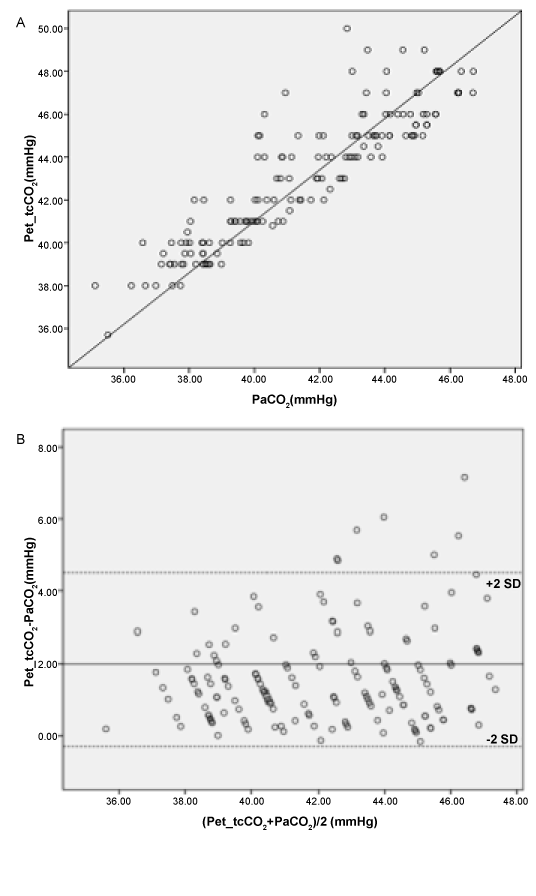Figure 4: (A) Correlation between PaCO2 and Pet_tcCO2 shows a coefficient R2=0.66. Regression analysis: PaCO2 = 0.83 × Pet_tcCO2+5.47. (B) Bias and LOA between PaCO2 and simultaneously measured Pet_tcCO2 measurements. The solid line represents the mean of the differences (= bias) between PaCO2 and PetCO2 (2.0 mmHg). The dashed lines are equivalent to 2SDs of the means (= LOA).